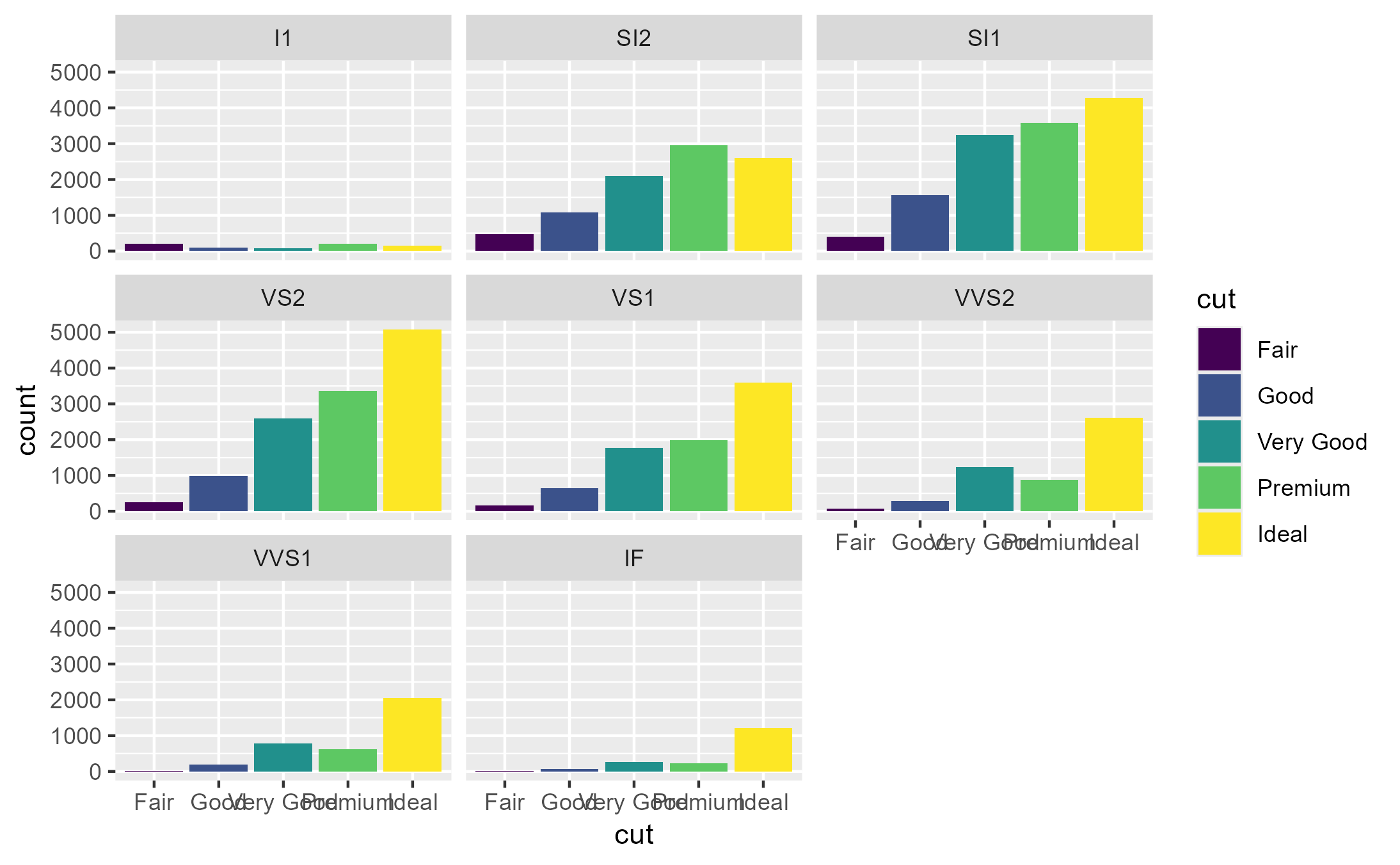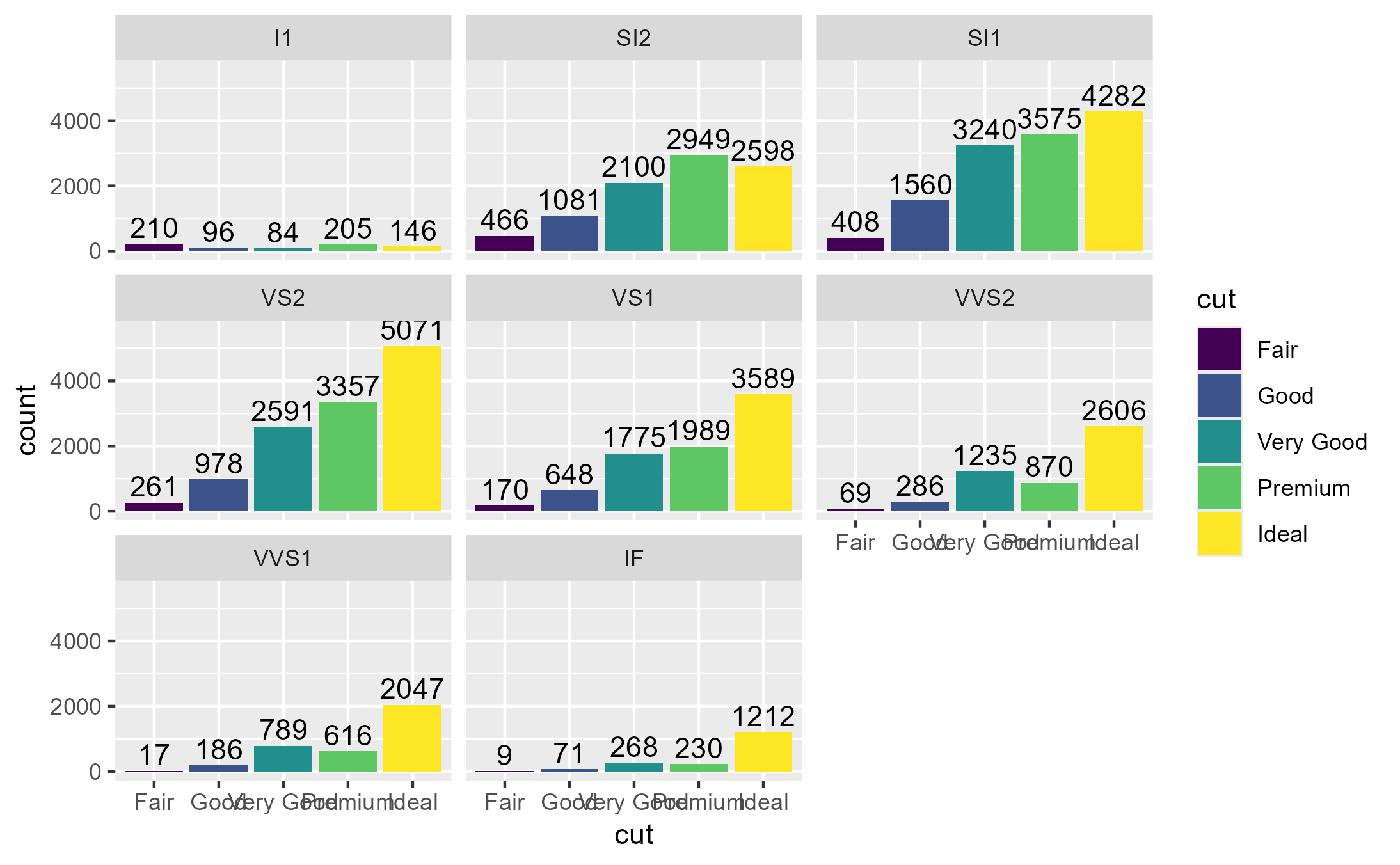Inspect which calls to a ggproto method met a particular condition

## Usage

ggtrace_inspect_which(x, method, cond, error = FALSE)

## Arguments

x

A ggplot object

method

A function or a ggproto method. The ggproto method may be specified using any of the following forms:

• ggproto$method • namespace::ggproto$method

• namespace:::ggproto$method cond Expression evaluating to a logical inside method when x is evaluated. error If TRUE, continues inspecting the method until the ggplot errors. This is useful for debugging but note that it can sometimes return incomplete output. ## Value The values of the tracing context variable ._counter_ when cond is evaluated as TRUE. ## Tracing context When quoted expressions are passed to the cond or value argument of workflow functions they are evaluated in a special environment which we call the "tracing context". The tracing context is "data-masked" (see rlang::eval_tidy()), and exposes an internal variable called ._counter_ which increments every time a function/method has been called by the ggplot object supplied to the x argument of workflow functions. For example, cond = quote(._counter_ == 1L) is evaluated as TRUE when the method is called for the first time. The cond argument also supports numeric shorthands like cond = 1L which evaluates to quote(._counter_ == 1L), and this is the default value of cond for all workflow functions that only return one value (e.g., ggtrace_capture_fn()). It is recommended to consult the output of ggtrace_inspect_n() and ggtrace_inspect_which() to construct expressions that condition on ._counter_. For highjack functions like ggtrace_highjack_return(), the value about to be returned by the function/method can be accessed with returnValue() in the value argument. By default, value is set to quote(returnValue()) which simply evaluates to the return value, but directly computing on returnValue() to derive a different return value for the function/method is also possible. ## Examples  library(ggplot2) p1 <- ggplot(diamonds, aes(cut)) + geom_bar(aes(fill = cut)) + facet_wrap(~ clarity) p1# Values of ._counter_ when compute_group is called for groups in the second panel: ggtrace_inspect_which(p1, StatCount$compute_group, quote(data$PANEL == 2)) #>  6 7 8 9 10 # How about if we add a second layer that uses StatCount? p2 <- p1 + geom_text( aes(label = after_stat(count)), stat = StatCount, position = position_nudge(y = 500) ) p2ggtrace_inspect_which(p2, StatCount$compute_group, quote(data$PANEL == 2)) #>  6 7 8 9 10 46 47 48 49 50 # Behaves like base::which() and returns integer(0) when no matches are found ggtrace_inspect_which(p2, StatBoxplot$compute_group, quote(data\$PANEL == 2))
#> integer(0)